# Use a graphing utility with vector capabilities to find u x v. u = (-4, 2,...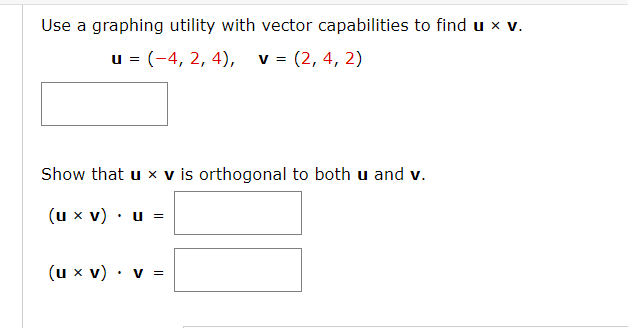Use a graphing utility with vector capabilities to find u x v. u = (-4, 2, 4), v = (2, 4, 2) Show that u x v is orthogonal to both u and v. (u x V) u = (u x V): V =

Using the graphing utility the result is

u × v= (-12, 16 , -20)

Now we find the other results.

(u × v) • u = 0

(u × v) • v = 0

Hence orthogonal property satisfied.

##### Add Answer of: Use a graphing utility with vector capabilities to find u x v. u = (-4, 2,...
Similar Homework Help Questions
• ### Linear Algebra - Find u x v

Use a graphing utility with vector capabilities to find u x v and then show that it is orthogonal to both u and vu = 3i - j + k,v = 2i + j - kThank you, please show work

• ### Linear Algebra - vector/orthogonal

Use a graphing utility with vector capabilities to find u x v and then show that it is orthogonal to both u and vu = (1,2, -3),v = (-1,1,2)Thank you, please show work

• ### Linear Algebra

Use a graphing utility with vector capabilities to find u x v and then show that it is orthogonal to both u and vu = (1,2, -3),v = (-1,1,2)Thank you, please show work

• ### Inner Product Spaces

Using a graphing utility with vector capabilities to find u xv and then show that it is orthogonal to both u and v.u = (0,1,-1), v = (1,2,0)

• ### Inner Product Spaces

Using a graphing utility with vector capabilities to find u xv and then show that it is orthogonal to both u and v.u = 2i + j - kv = i - j + 2k

• ### Exercise Set Chapter 3 Q1) Let u = (2, -2, 3), v = (1, -3, 4),...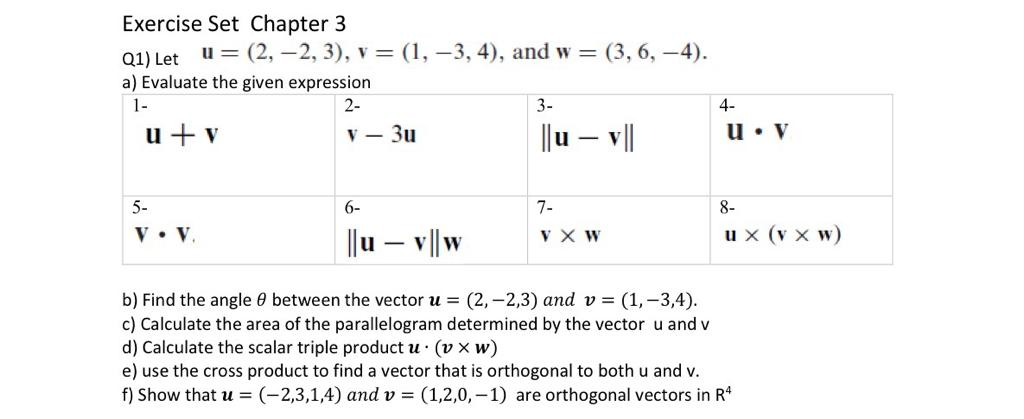Exercise Set Chapter 3 Q1) Let u = (2, -2, 3), v = (1, -3, 4), and w=(3,6,-4). a) Evaluate the given expression u + v V - 3u ||u – v| u. V lju – v|w V X W ux (v x W) b) Find the angle 8 between the vector u = (2,-2,3) and v = (1, -3,4). c) Calculate the area of the parallelogram determined by the vector u and v d) Calculate the scalar triple product...

• ### ra Use a graphing utility to complete the table and estimate the limit as x approaches infinity. Then use a g f (x...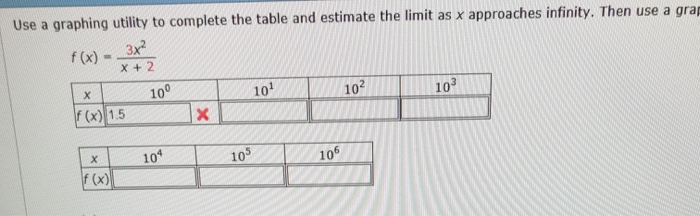ra Use a graphing utility to complete the table and estimate the limit as x approaches infinity. Then use a g f (x)--3x2- x + 2 10 10 10 10 f (x) 1.5 10 10 T | 29,4| 42.6| 50.5 5aj 58.9 75 90 1051 120 63,0 66,4| 67.3 | 60.0 for the data(Round your coeficents to hee (a) Use the regression capabilities of a graphing ulity to find a model of the form T,-tbe b) Use a graphing utlty...

• ### Find a unit vector orthogonal to both u and v. u = i - 2j V...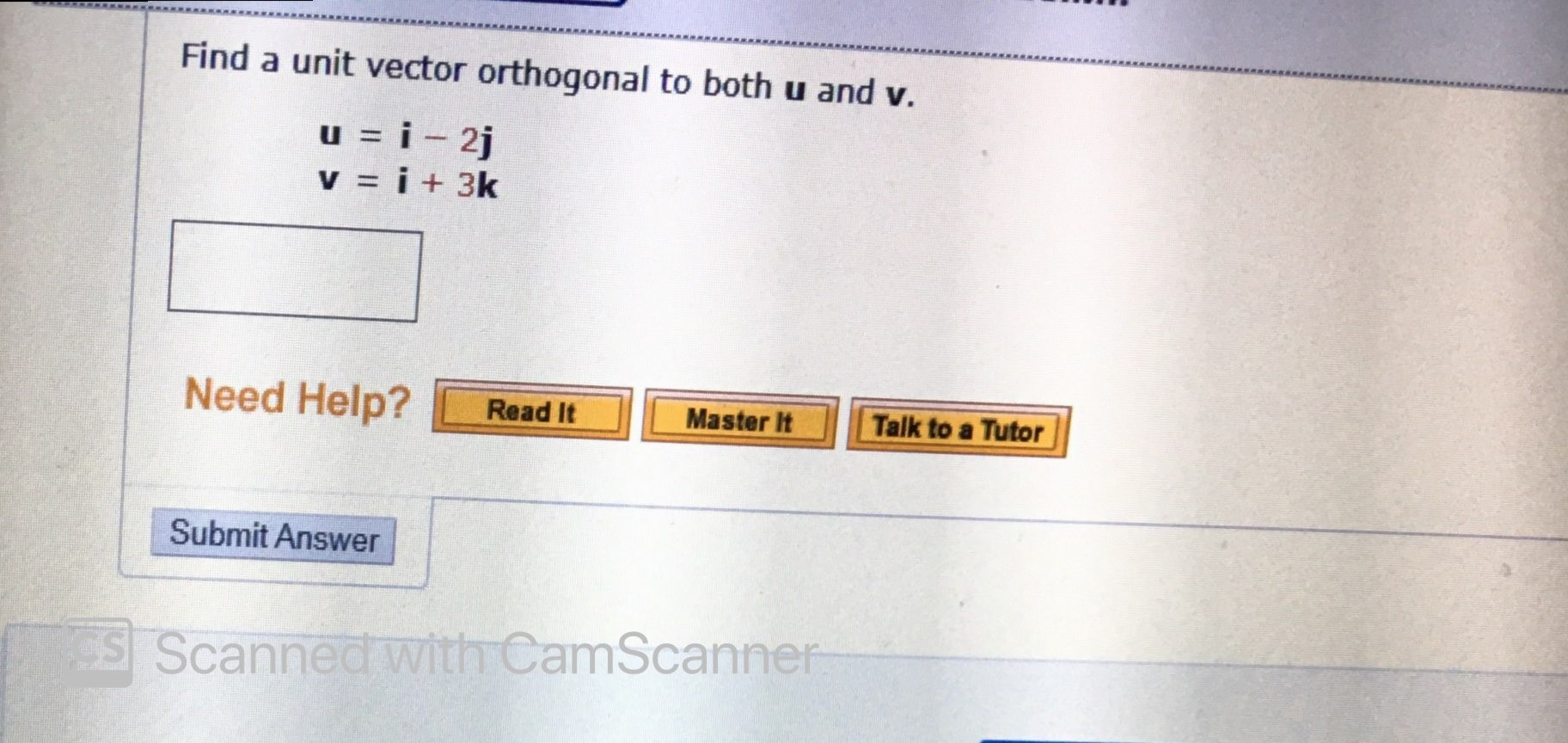Find a unit vector orthogonal to both u and v. u = i - 2j V = i + 3k Need Help? Read It Master It Talk to a Tutor Submit Answer Scanned with CamScanner

• ### USE MATLAB TO ANSWER PLEASE Let u = | 2 | and v = . Use the MATLAB functions normo, cross(), and dot() , to complete th...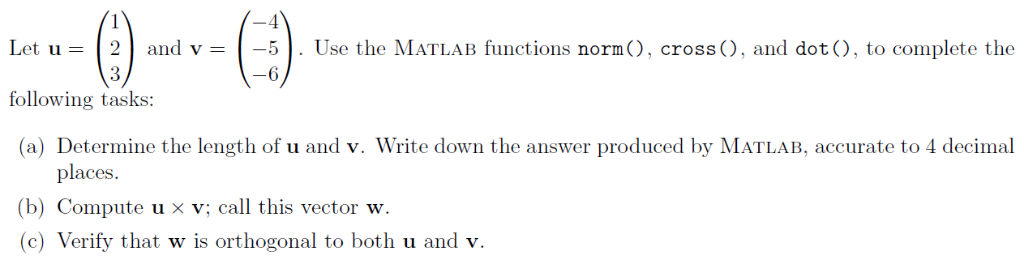USE MATLAB TO ANSWER PLEASE Let u = | 2 | and v = . Use the MATLAB functions normo, cross(), and dot() , to complete the -6 following tasks: (a) Determine the length of u and v. Write down the answer produced by MATLAB, accurate to 4 decimal places (b) Compute u x v; call this vector w. (c) Verify that w is orthogonal to both u and v Let u = | 2 | and v = ....

• ### (Section 11.3) Find the projection of u onto v and find the vector component of u...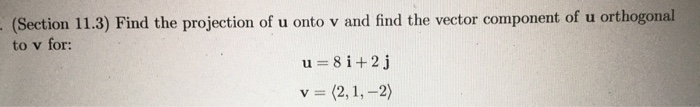(Section 11.3) Find the projection of u onto v and find the vector component of u orthogonal to v for: u=8 i+2j v = (2, 1, -2)

Free Homework App